# Uniform star polyhedron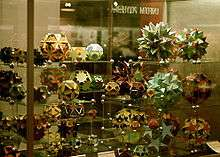A display of uniform polyhedra at the Science Museum in London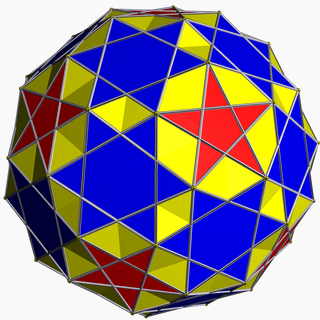The small snub icosicosidodecahedron is a uniform star polyhedron, with vertex figure 35.5/2

In geometry, a uniform star polyhedron is a self-intersecting uniform polyhedron. They are also sometimes called nonconvex polyhedra to imply self-intersecting. Each polyhedron can contain either star polygon faces, star polygon vertex figures or both.

The complete set of 57 nonprismatic uniform star polyhedra includes the 4 regular ones, called the Kepler–Poinsot polyhedra, 5 quasiregular ones, and 48 semiregular ones.

There are also two infinite sets of uniform star prisms and uniform star antiprisms.

Just as (nondegenerate) star polygons (which have Polygon density greater than 1) correspond to circular polygons with overlapping tiles, star polyhedra that do not pass through the center have polytope density greater than 1, and correspond to spherical polyhedra with overlapping tiles; there are 48 nonprismatic such uniform star polyhedra. The remaining 9 nonprismatic uniform star polyhedra, those that pass through the center, are the hemipolyhedra, and do not correspond to spherical polyhedra, as the center cannot be projected uniquely onto the sphere.

The nonconvex forms are constructed from Schwarz triangles.

All the uniform polyhedra are listed below by their symmetry groups and subgrouped by their vertex arrangements.

Regular polyhedra are labeled by their Schläfli symbol. Other nonregular uniform polyhedra are listed with their vertex configuration or their Uniform polyhedron index U(1-80).

Note: For nonconvex forms below an additional descriptor Nonuniform is used when the convex hull vertex arrangement has same topology as one of these, but has nonregular faces. For example an nonuniform cantellated form may have rectangles created in place of the edges rather than squares.

## Tetrahedral symmetry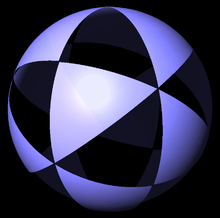(3 3 2) triangles on sphere

There is one nonconvex form, the tetrahemihexahedron which has tetrahedral symmetry (with fundamental domain Möbius triangle (3 3 2)).

There are two Schwarz triangles that generate unique nonconvex uniform polyhedra: one right triangle (3/2 3 2), and one general triangle (3/2 3 3). The general triangle (3/2 3 3) generates the octahemioctahedron which is given further on with its full octahedral symmetry.

Vertex arrangement
(Convex hull)
Nonconvex forms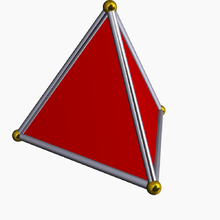Tetrahedron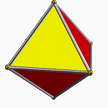Rectified tetrahedron
Octahedron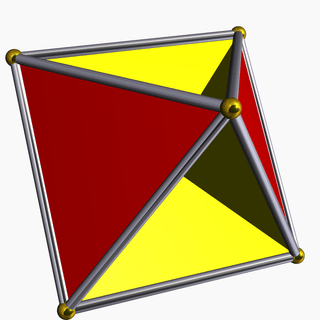(4.3/2.4.3)
3/2 3 | 2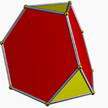Truncated tetrahedron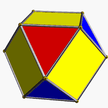Cantellated tetrahedron
(Cuboctahedron)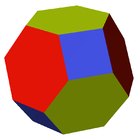Omnitruncated tetrahedron
(Truncated octahedron)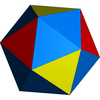Snub tetrahedron
(Icosahedron)

## Octahedral symmetry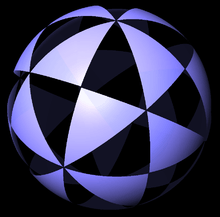(4 3 2) triangles on sphere

There are 8 convex forms, and 10 nonconvex forms with octahedral symmetry (with fundamental domain Möbius triangle (4 3 2)).

There are four Schwarz triangles that generate nonconvex forms, two right triangles (3/2 4 2), and (4/3 3 2), and two general triangles: (4/3 4 3), (3/2 4 4).

Vertex arrangement
(Convex hull)
Nonconvex forms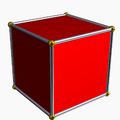Cube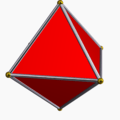Octahedron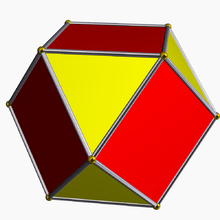Cuboctahedron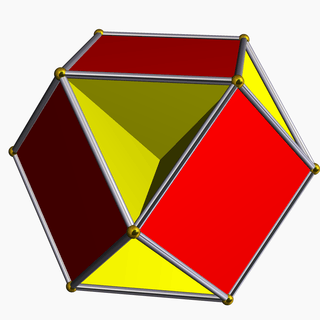(6.4/3.6.4)
4/3 4 | 3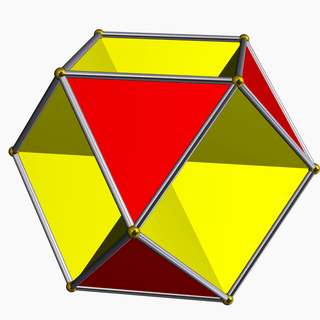(6.3/2.6.3)
3/2 3 | 3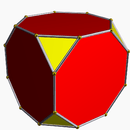Truncated cube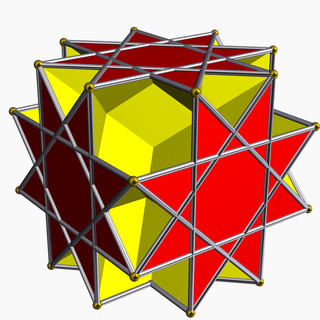(4.8/3.4/3.8/5)
2 4/3 (3/2 4/2) |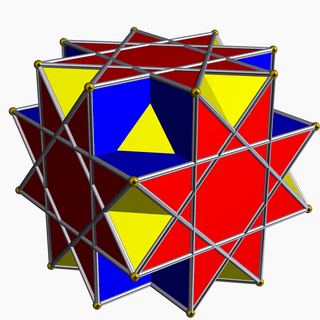(8/3.3.8/3.4)
3 4 | 4/3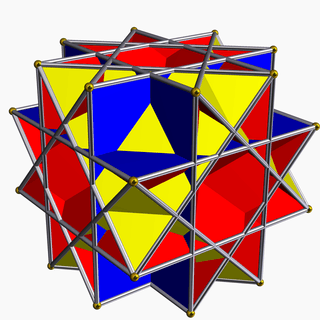(4.3/2.4.4)
3/2 4 | 2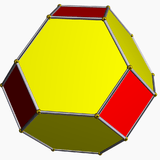Truncated octahedron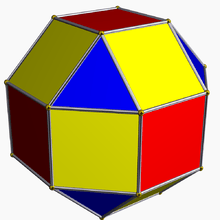Rhombicuboctahedron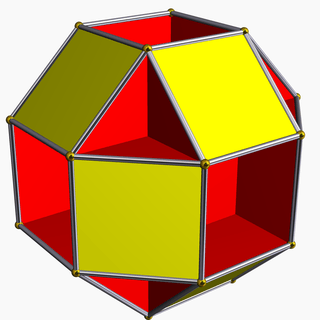(4.8.4/3.8)
2 4 (3/2 4/2) |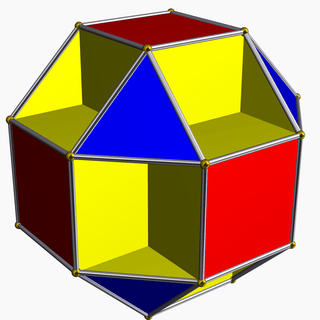(8.3/2.8.4)
3/2 4 | 4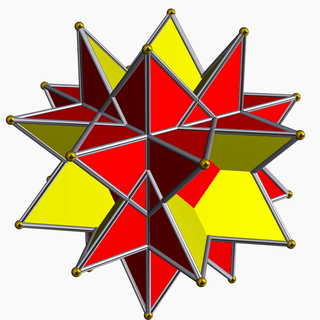(8/3.8/3.3)
2 3 | 4/3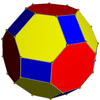Nonuniform
truncated cuboctahedron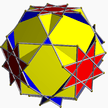(4.6.8/3)
2 3 4/3 |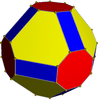Nonuniform
truncated cuboctahedron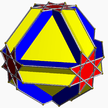(8/3.6.8)
3 4 4/3 |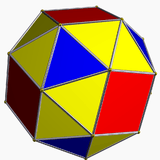Snub cube

## Icosahedral symmetry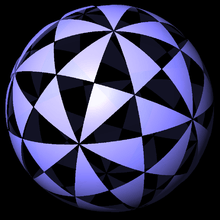(5 3 2) triangles on sphere

There are 8 convex forms and 46 nonconvex forms with icosahedral symmetry (with fundamental domain Möbius triangle (5 3 2)). (or 47 nonconvex forms if Skilling's figure is included). Some of the nonconvex snub forms have reflective vertex symmetry.

Vertex arrangement
(Convex hull)
Nonconvex forms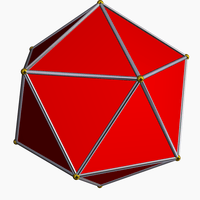Icosahedron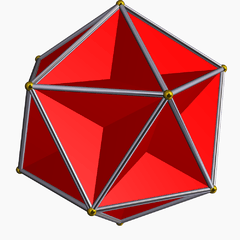{5,5/2}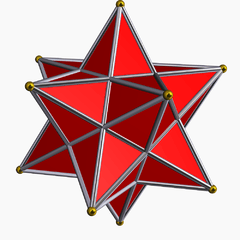{5/2,5}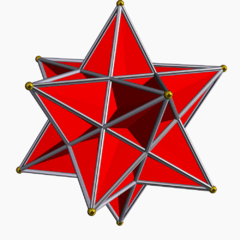{3,5/2}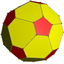Nonuniform
truncated icosahedron
2 5 |3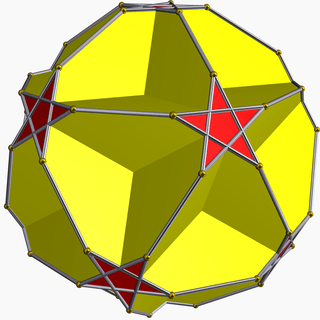U37
2 5/2 | 5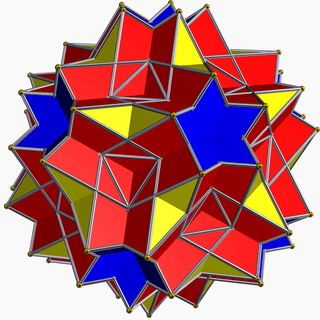U61
5/2 3 | 5/3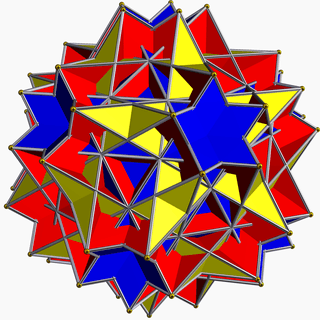U67
5/3 3 | 2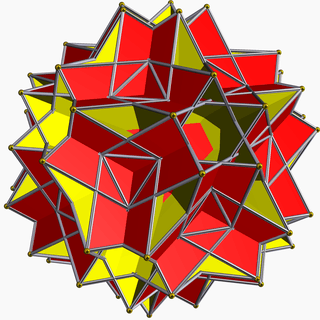U73
2 5/3 (3/2 5/4) |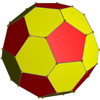Nonuniform
truncated icosahedron
2 5 |3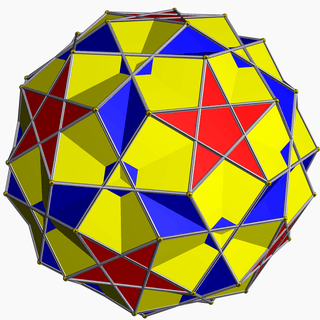U38
5/2 5 | 2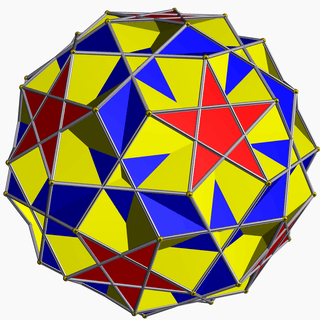U44
5/3 5 | 3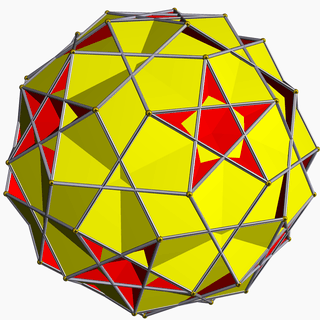U56
2 3 (5/4 5/2) |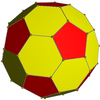Nonuniform
truncated icosahedron
2 5 |3U32
| 5/2 3 3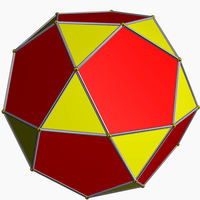Icosidodecahedron
2 | 3 5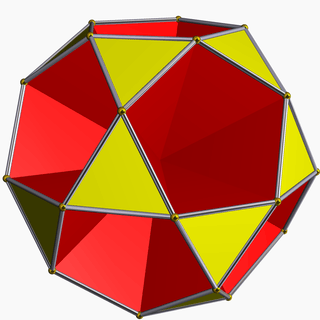U49
3/2 3 | 5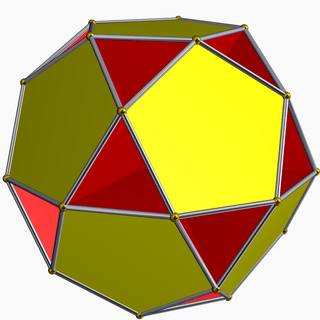U51
5/4 5 | 5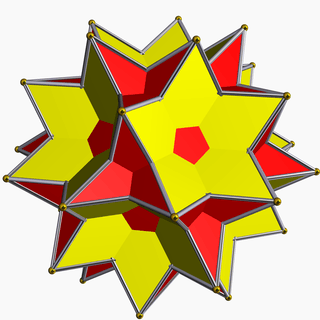U54
2 | 3 5/2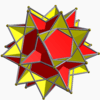U70
5/3 5/2 | 5/3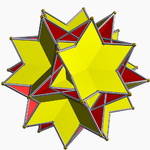U71
3 3 | 5/3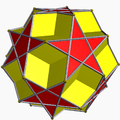U36
2 | 5 5/2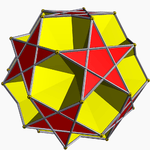U62
5/3 5/2 | 3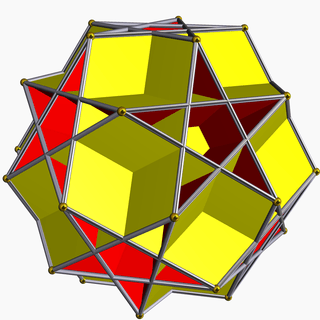U65
5/4 5 | 3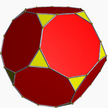Truncated dodecahedron
2 3 | 5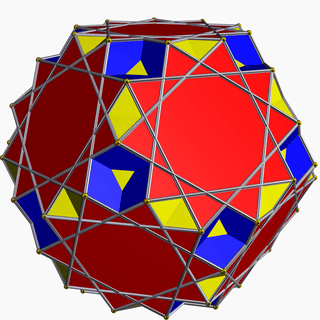U42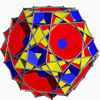U48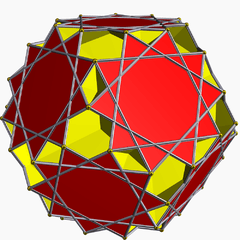U63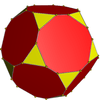Nonuniform
truncated dodecahedron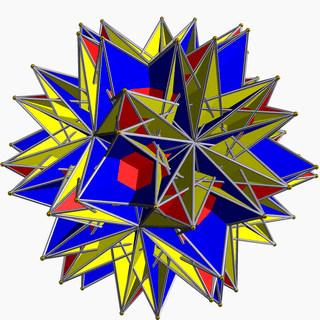U72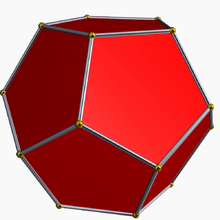Dodecahedron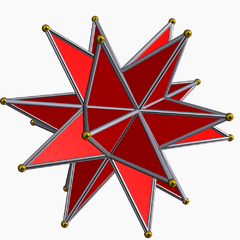{5/2,3}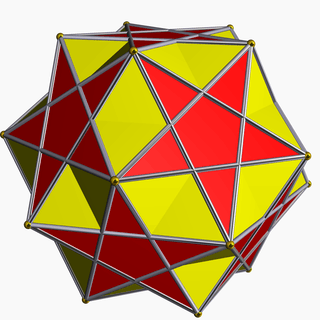U30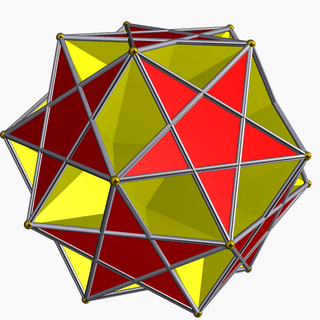U41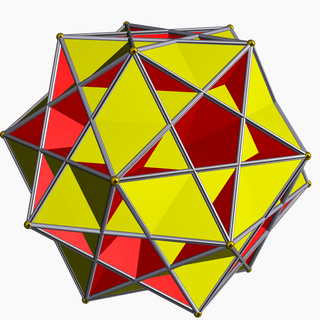U47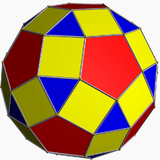Rhombicosidodecahedron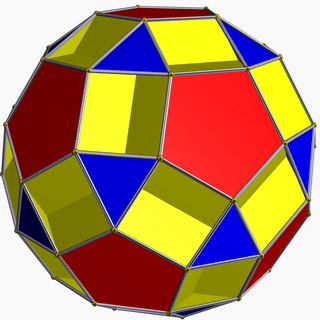U33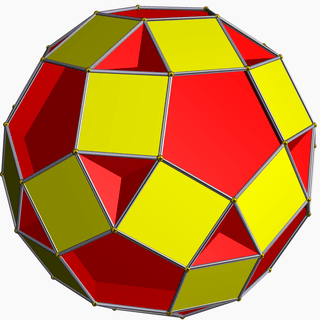U39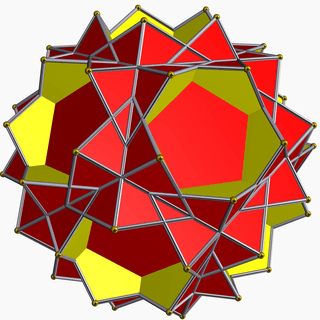U58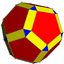Beveled
Dodecahedron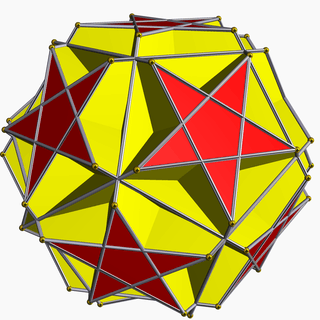U55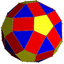Nonuniform
rhombicosidodecahedron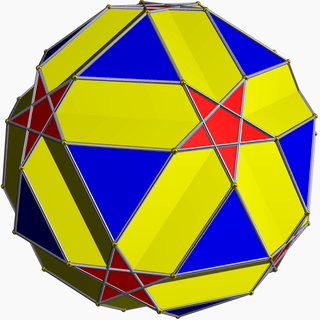U31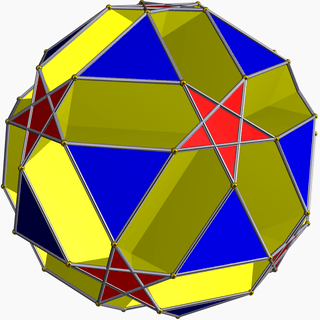U43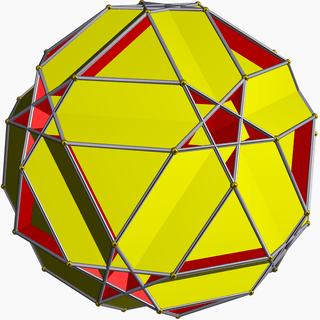U50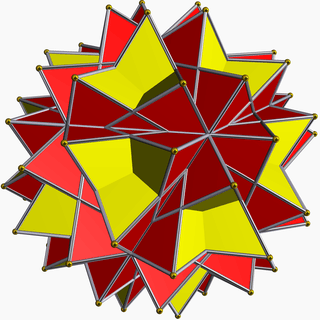U66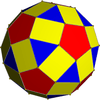Nonuniform
rhombicosidodecahedron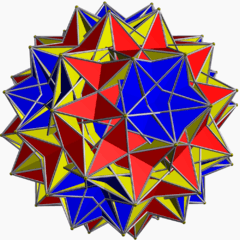U75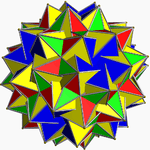U64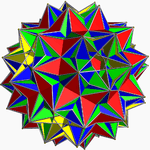Skilling's figure
(see below)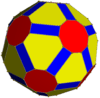Nonuniform
truncated icosidodecahedron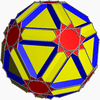U45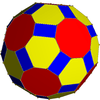Nonuniform
truncated icosidodecahedron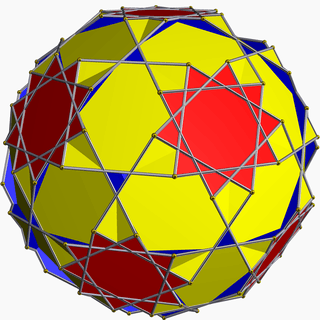U59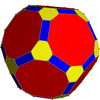Nonuniform
truncated icosidodecahedron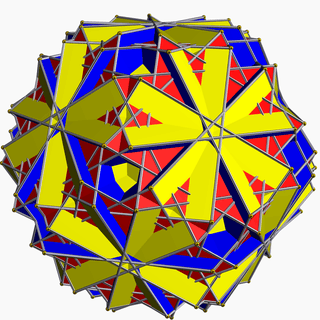U68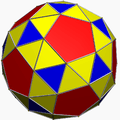Nonuniform
snub dodecahedron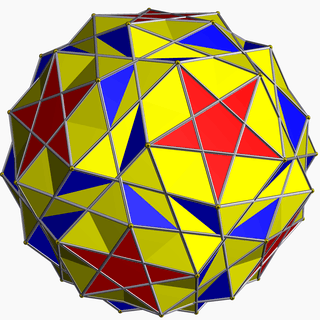U40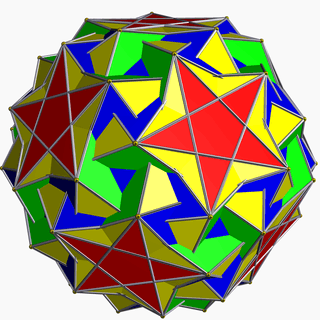U46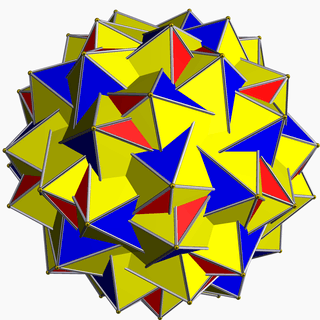U57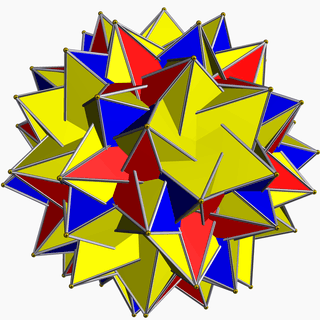U69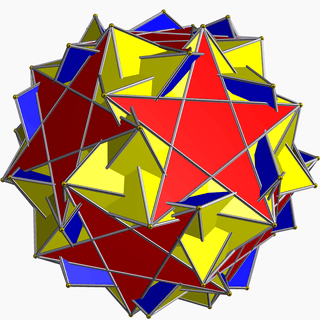U60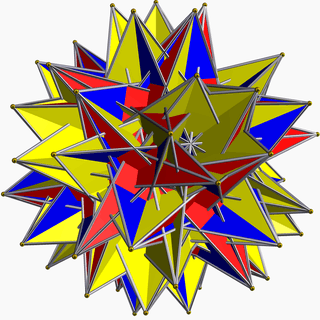U74

## Degenerate cases

Coxeter identified a number of degenerate star polyhedra by the Wythoff construction method, which contain overlapping edges or vertices. These degenerate forms include:

### Skilling's figure

One further nonconvex degenerate polyhedron is the Great disnub dirhombidodecahedron, also known as Skilling's figure, which is vertex-uniform, but has pairs of edges which coincide in space such that four faces meet at some edges. It is counted as a degenerate uniform polyhedron rather than a uniform polyhedron because of its double edges. It has Ih symmetry.